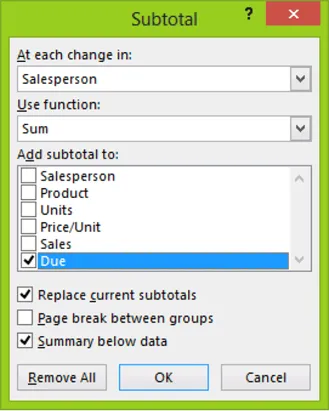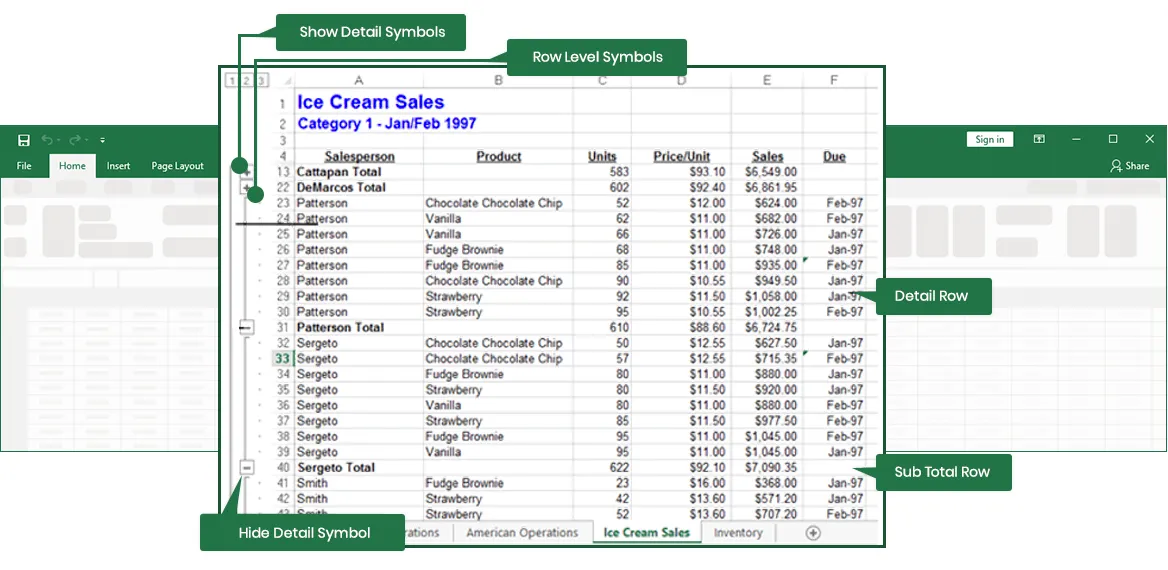# Using Subtotals in an Excel List

When working with a list, you often need to know the bottom line figures or totals. A list of sales records may include the names of all salespersons, the different products they have sold, and the units of each product sold. To get a better idea of each person’s performance by product, you can use the Subtotals command to get a subtotal for each product sold by each salesperson.

#### Using Automatic Subtotals

Inserting automatic subtotals is a quick way to summarize data in a list. To use automatic subtotals, your data must be organized into a list and sorted by the column for which you want to display subtotals. For example, in a list of sales records, you sort by salesperson to obtain the subtotals for the number of product units sold by each salesperson.

You determine how you want to subtotal your data using the Subtotal dialog box, shown in Figure 1-19. The options available in the Subtotal dialog box are summarized in Table 1-1.

For hands-on Microsoft Excel training classes in Los Angeles call us on 888.815.0604.Figure 1-19: The Subtotal Dialog Box

 At Each Change In Function At Each Change In Identifies the field that contains the items by which you want to subtotal values in other columns. Use Function Identifies the summary function used to calculate subtotals. Add Subtotal To Identifies the fields you wish to subtotal. Replace Current Subtotals Displays new subtotal rows. Page Break Between Groups Inserts a page break between each grouping. Summary Below Data Adds subtotal rows below the groupings.

Table 1-1: Subtotal Dialog Box Options

Excel calculates subtotal values by using a summary function, such as Sum or Average. The grand total values are derived from the detail data, which is the list data, and not from the subtotal rows. Grand total values are calculated by using the same summary function that you use to calculate subtotal values. Both grand total and subtotal labels are displayed in bold in the list and are automatically recalculated as you edit detail rows.

#### Method

###### To display automatic subtotals:
1. Sort the list by the column for which you want to display subtotals.
2. If necessary, select a cell in the list.
3. In the Outline group on the Data tab, click the Subtotal button.
4. In the Subtotal dialog box, from the At each change in drop‑down list, select a field.
5. From the Use function drop-down list, select a subtotal function.
6. In the Add subtotal to list box, select the desired subtotal field check box(es).
7. If necessary, select the Replace current subtotals and Summary below data check boxes, and deselect the Page break between groups check box.
8. Choose OK.
###### To remove automatic subtotals:
1. In the Outline group on the Data tab, click the Subtotal button.
2. In the Subtotal dialog box, choose Remove All.

#### Exercise

###### In the following exercise, you will use automatic subtotals in a list.
1. Select the Ice Cream Sales worksheet.
2. Sort the list first by Salesperson and then by Product, both in ascending order.
3. In the Outline group on the Data tab, click the Subtotal button. [The Subtotal dialog box appears].
4. In the At each change in drop-down list box, make sure Salesperson is selected.
5. In the Use function drop-down list box, make sure Sum is selected.
6. In the Add Subtotal to list box, select the Units, Price/Unit, and Sales check boxes, deselecting other check boxes as necessary.
7. Make sure the Replace current subtotals and Summary below data check boxes are selected, and the Page break between groups check box is deselected.
8. Choose OK. [Automatic subtotals are inserted for each salesperson].

#### Working with Subtotal Outline Symbols

When you use automatic subtotals, various subtotal outline symbols are displayed to the left of the worksheet, as illustrated in Figure 1-20. You click the appropriate detail symbols to hide or show rows of detail data. Excel automatically outlines your list by grouping detail rows with each associated subtotal row and by grouping subtotal rows with the grand total row.

You can also hide or show details for all subtotal groups at once by using the row level symbols. The level 1 symbol hides all levels of data except the grand total rows. The level 2 symbol hides all levels of data except the subtotal and grand total rows. The level 3 symbol shows all the data.Figure 1-20: Subtotal Outline Symbols

#### Method

###### To hide the detail rows of an automatic subtotaled list:
1. Click the hide detail symbol beside the subtotal group.
2. To show the detail rows of an automatic subtotaled list:
3. Click the show detail symbol beside the subtotal group.
4. To show different levels of detail in an automatic subtotaled:
5. Click the appropriate level symbol.

#### Exercise

###### In the following exercise, you will work with subtotal outline symbols.
1. To the left of row 13, click the hide detail symbol for Cattapan Total. [The detail data for Cattapan is hidden. Only the subtotal row for Cattapan is visible].
2. To the left of row 22, click the hide detail symbol for DeMarcos Total. [The detail data for DeMarcos is hidden. Only the subtotal row for DeMarcos is visible].
3. Click the row level 2 symbol. [Only the subtotal rows in the list are displayed].
4. Click the row level 1 symbol. [Only the grand total row is displayed].
5. To the left of row 59, click the show detail symbol for Grand Total. [The subtotal rows reappear].
6. Click the row level 3 symbol. [All detail data, and the subtotal and grand total rows, are now visible].

#### Adding and Deleting Records Using the Data Form

If the existing subtotals do not show enough detail, you can insert a subtotal within a subtotal group, called a nested subtotal. For example, if your list shows the total number of products sold for each salesperson, you can add further detail to the list by adding subtotals for each individual product.

#### Method

###### To nest subtotals:
1. In the Outline group on the Data tab, click the Subtotal button.
2. In the Subtotal dialog box, from the At each change in drop-down list, select the field you want to use for the nested subtotal grouping.
3. From the Use function drop-down list and in the Add subtotal to list box, select any new options for the new subtotal.
4. Deselect the Replace current subtotals check box.
5. Choose OK.

#### Exercise

###### In the following exercise, you will nest subtotals.
1. In the Outline group on the Data tab, click the Subtotal button. [The Subtotal dialog box appears].
2. From the At each change in drop-down list, select Product.
3. Deselect the Replace current subtotals check box. [The Summary below data option is dimmed].
4. Choose OK. [The Subtotal dialog box closes, and the new nested subtotals appear. A new row level symbol, 4, appears].
5. Click the row level 3 symbol. [The detail data is hidden].
6. Open the Subtotal dialog box.
7. Choose Remove All, and then save and close the workbook. [All subtotals and outline symbols are removed].

#### Excel training testimonials

We train thousands of students to use Excel every year. To read a sample of our reviews please click the following link Excel testimonials.